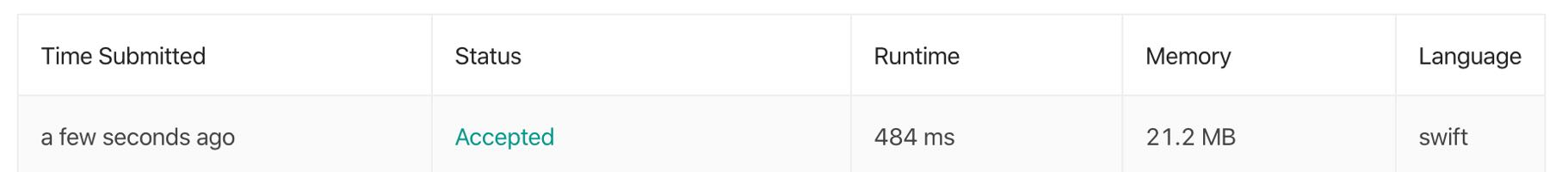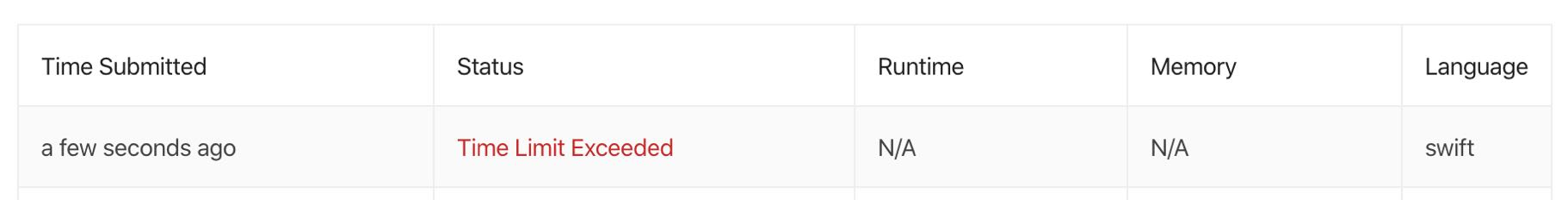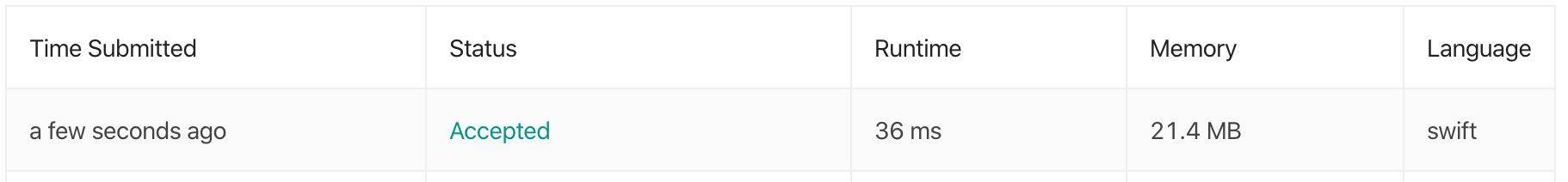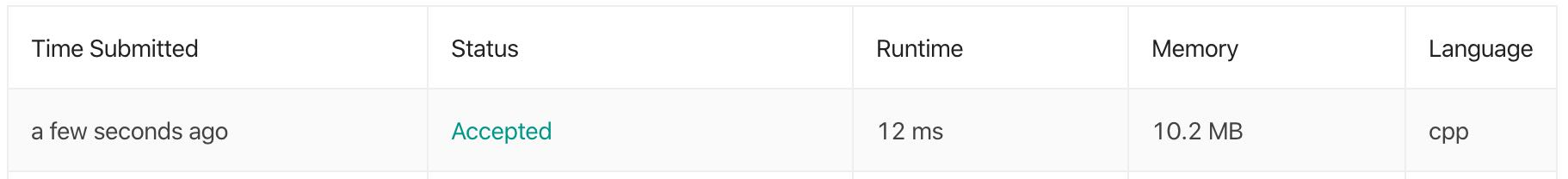# How much interesting the simplest leetcode problem could be?

Even the simplest Leetcode problem could teach you a lot. And this is my story.

## Problem

Let's checkou the problem first.

Given an array of integers, return indices of the two numbers such that they add up to a specific target.

You may assume that each input would have exactly one solution, and you may not use the same element twice.

``````Given nums = [2, 7, 11, 15], target = 9,

Because nums + nums = 2 + 7 = 9,
return [0, 1].
``````

## Principle

During the solving and optimizing procedure, make sure you have utilized ALL the conditions provided by the problem. Yes, all of them.

## Brute Force

If you don't know much about the problem, the best way of solving the problem is by writing some code intuitively, especially for this kind of simple one.

So my first try is just a Swift translation of the problem:

``````/// Solution 1
func twoSum(_ nums: [Int], _ target: Int) -> [Int] {
var i = 0
var j = i + 1

for n in i ..< nums.count - 1 {
for m in j ..< nums.count {
if nums[n] + nums[m] == target {
return [n, m]
}
}

i += 1
j = i + 1
}

return [0, 0]
}
``````

The implementation is quite straightforward. We add `nums[n]` with each element in the rest of `num`. If the sum equals `target`, return `[n, m]` directly, or return `[0, 0]` for an empty match (The problem do not specify how to handle errors).

• The time complexity of this brute force approach is `O(n^2)`;
• The space complexity is `O(1)`;

If we try to submit this answer to Leetcode, we will get the following result:Every experienced programmer knows that an `O(n^2)` algorithm should be avoided.

## Optimize the complement query process

In order to optimize the algorithm, we need to understand why its time complexity is `O(n^2)`: When trying to find the complement of `num[n]`, we need to iterate through the rest of `num` which is an `O(n)` process. If we can optimize it to `O(1)`, the whole time complexity will down to `O(n)`.

As we know, fetch elements from a dictionary is an `O(1)` algorithm. So this is my first optimization:

``````func twoSum(_ nums: [Int], _ target: Int) -> [Int] {
let lookup: [Int: Int] = Dictionary(uniqueKeysWithValues: nums.enumerated())

for (i, v) in nums.enumerated() {
if let (index, _) = lookup.first { \$0.1 + v == target } else {
return [i, index] }
}
}

return [0, 0]
}
``````

Quite understandable, right? I try to build a `Dictionary` which is keyed by each index of the element of `num`, then traverse `num` and find a possible complement through `lookup`. But unfortunately, this would not compile and I got serveral compile errors which make me know more about Swift.

### A bug of EnumeratedSequence

The first, `Dictionary(uniqueKeysWithValues: nums.enumerated())` won't compile. The compiler complains that: Generic parameter 'Key' could not be inferred. But even if you specifiy the `Key` and `Value` explicitly:

``````Dictionary<Int, Int>(uniqueKeysWithValues: nums.enumerated())
``````

You will get a more wiered error: Generic parameter 'S' could not be inferred. Emm... Actually, I don't know what's the the generic parameter `S`. But after googling this problem, I found a thread on on official Swift forum and a bug of SR-6305 talking about this problem.

Simply speaking, the core problem is that the element type of `EnumeratedSequence`, which is returned by `enumerated`, is a tuple with tags like this:

``````typealias Element = (offset: Int, element: Base.Element)
``````

This is why `Dictionary<Key, Value>(uniqueKeysWithValues:)` cannot extract values from it to build a `Dictionary`. The interesting here is that the following code works:

``````let r1 = [(offset: 0, element: 1), (offset: 1, element: 2)]
let dict1 = Dictionary(uniqueKeysWithValues: r1) // [1:2, 0:1]
``````

But this failed:

``````let r2 = [1, 2].enumerated()
let dict2 = Dictionary(uniqueKeysWithValues: r2)
// Error: Generic parameter 'Key' could not be inferred
``````

Currently, the workaround is map `r2` to an `[(offset: Int, element: Int)]`:

``````let r2 = [1, 2].enumerated().map { \$0 }
let dict2 = Dictionary(uniqueKeysWithValues: r2) // [1:2, 0:1]
``````

But actually, I don't like this workaround, it's verbose. Since the `EnumeratedSequence` doesn't work, we can build a similar data structure by `zip`

``````let r3 = zip(0..., [1, 2])
let dict3 = Dictionary(uniqueKeysWithValues: r3)
``````

And it looks much better.

### Cannot use trailing closure syntax in a `if let` expression

This is my second optimization:

``````func twoSum(_ nums: [Int], _ target: Int) -> [Int] {
let lookup: [Int: Int] = Dictionary(uniqueKeysWithValues: zip(0..., nums))

for (i, v) in nums.enumerated() {
if let (index, _) = lookup.first { \$0.1 + v == target } else {
return [i, index] }
}
}

return [0, 0]
}
``````

And I failed again because we cannot use trailing closure syntax inside a `if let` expression. Compiler will match the first `{` as the open brace for `if let`, not the `first` function. You have to put it inside the calling:

``````for (i, v) in nums.enumerated() {
if let (index, _) = lookup.first(where: { \$0.1 + v == target }) else {
return [i, index] }
}
}
``````

But I like the trailing closure style and update the `for` loop like this:

``````for (i, v) in nums.enumerated() {
let elem = lookup.first { \$0.value == (target - v) }
if let (index, _) = elem { return [i, index] }
}
``````

If you submit this to Leetcode, you will be blocked by a testcase like this:

``````twoSum([3, 2, 4], 6)
``````

The expected result is `[1, 2]`, but our implementation returns `[0, 0]`. This is because `3 + 3 = 6` and the index returned by `lookup.first { \$0.value == (target - v) }` is exactly the same as the element we currently traversed. This is why the problem requires that you may not use the same element twice. So except for checking `elem` is not `nil`, we have to check the `index` is not equal to `i`. And this is my semi final version:

``````func twoSum(_ nums: [Int], _ target: Int) -> [Int] {
let lookup: [Int: Int] = Dictionary(uniqueKeysWithValues: zip(0..., nums))

for (i, v) in nums.enumerated() {
let elem = lookup.first { \$0.value == (target - v) }
if let (index, _) = elem, index != i { return [i, index] }
}

return [0, 0]
}
``````

But after submitting to Leetcode, I failed again. Yes again! My implementation was out of time limiting. The only explanation might be creating a `Dictionary` from a zipped sequence is not as efficient as I expected.But the initial direction is still right. We need to reduce the time complexity of the complement number query.

What can we do next?

## Final optimization

The idea is we can combine the first two version of `twoSum`. While we iterate `num`, we add the element into `lookup`. We also check if the complement is already in the `lookup`. If it exists, we get the answer immediately:

``````func twoSum(_ nums: [Int], _ target: Int) -> [Int] {
var lookup: [Int: Int] = [:]

for (i, v) in nums.enumerated() {
if let index = lookup[target - v] {
return [index, i]
}

lookup[v] = i
}

return [0, 0]
}
``````

Submit this to Leetcode, and it's improved greatly:## Swift vs CPP

At the end of this article, let compare C++ version of the same algorithm against Swift:

``````vector<int> twoSum(vector<int>& nums, int target) {
map<int, int> record;

for (int i = 0; i < nums.size(); ++i) {
int complement = target - nums[i];
auto pos = record.find(complement);

if (pos != record.end()) {
return vector { pos->second, i };
}

record[nums[i]] = i;
}

return vector<int> {};
}
``````

And it's three times faster and half of memory requirement than Swift:## Things to remember

Write them down inside a card and it will help you recap the problem very quickly:

• Write some code intuitively first, do try to make every thing perfect at a single shot;
• Make sure you have check ALL the conditions and requirements the problem provided;
• Optimizing an `O(n^2)` algorithm could be started by reduce the time complexity of a inner loop;
• Matching a sum of `m + n` is just another phase of finding the complement of `m`;
• You cannot use trailing closure syntax inside a `if let` expression in Swift;
• Swift is still far more slow and expensive than C++ (LoL, just for fun, it doesn't mean C++ is better than Swift);